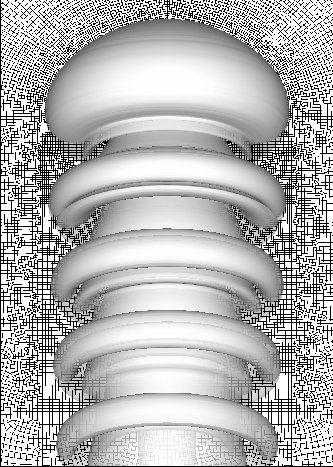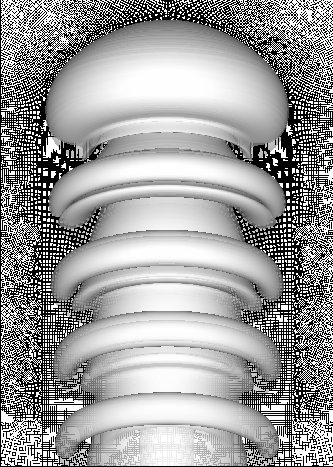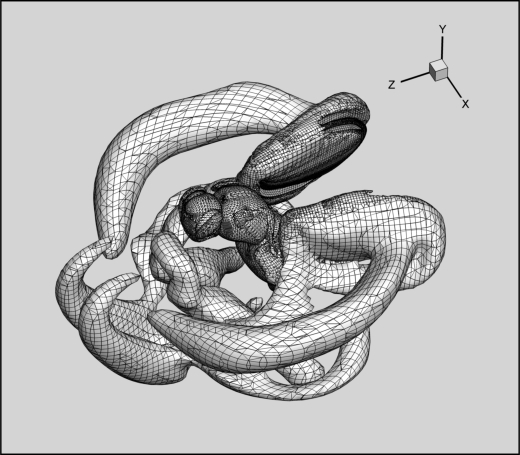THE NUMERICAL INVESTIGATION OF NEAR WAKE STRUCTURES FOR ANIMAL LOCOMOTIONS

A parallel large-scale unstructured finite volume algorithm based on an  arbitrary Lagrangian-Eulerian (ALE) formulation has been developed in order to investigate the near wake structure of the swimming/flying animals. In the ALE method, the mesh follows the interface between the fluid and solid boundary and the governing equations are discretized on unstructured moving meshes. This differs from the standard Eulerian formulation in a way that the mesh movement has to fulfill special conditions in order to maintain the accuracy and the stability of the time integration scheme. This condition is satisfied by the enforcement of the so-called discrete geometric conservation law (DGCL). The mesh deformation algorithm is based on either the indrect implementaion of radial basis function (RBF) or the solution of the elasticity equations.  For the parallel solution of resulting large-scale algebraic equations in a fully coupled form, a matrix factorization is introduced similar to that of the projection method for the whole system and two-cycle of BoomerAMG solver is used for the scaled discrete Laplacian provided by the HYPRE library which we access through the PETSc library.The numerical algorithm is used to study the time-variation  of the Eulerian and Lagrangian chorent structures in the near wake.Three dimensional wake structure behind a free-swimming hydromedusa Aequorea victoria (crystal jelly).Three dimensional near wake structure around the flapping Drosophila
(E. Dilek and B.Erzincanli).